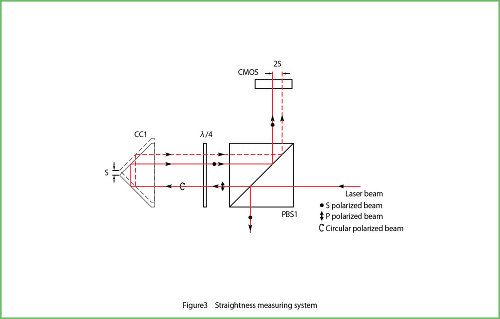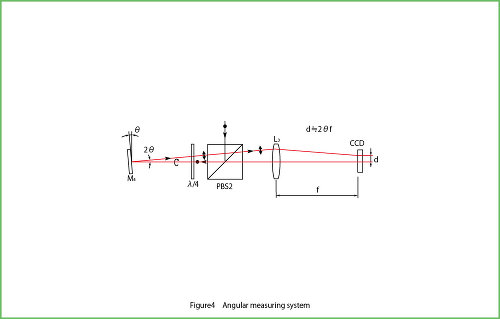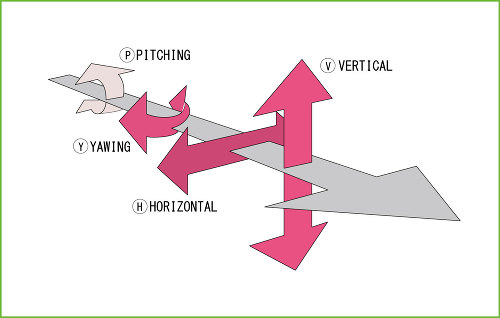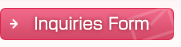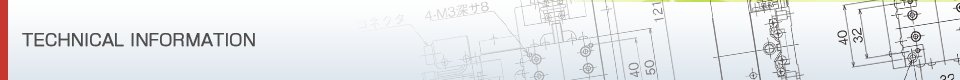## Measuring Instruments & Others

#### Measurement principle of straightor - Optical system

Figure 1 shows the optical system.
The straightener is the one-piece unit which consists of a straightness displacement measurement system making use of the corner cube prism's property and an angle displacement measurement system utilizing the auto collimator's principle.
The laser beam is linearly-polarized light that is inclined at 45°toward paper surface, which in turn becomes a parallel beam giving rise to less noise because of the beam expander.
Then, the beam which is reflected by the half mirror (HM) enters the image sensor 1. This is designed to detect a displacement of laser beam itself which may be caused by heat generation from laser, etc.
Moreover, the transmitted beam will be split into the polarized light beam P and beam S by means of the polarizing beam splitter (PBS1), which are perpendicular to each other in a horizontal direction and a vertical direction, respectively, with respect to the paper surface.
The transmitted polarized light beam P is used for straightness displacement measurement, and the transmitted polarized light beam S is used for angle displacement measurement. First, as shown in Figure 2, the polarized light beam P penetrates the PBS1, passes through 1/4 wavelength plate and becomes a circularly polarized light from a linearly polarized light. Second, the beam is reflected by the corner cube prism (CC1) which is mounted on an object to be measured, then becomes the polarized light beam S after passing through the 1/4 wavelength plate again.
It finally, in turn, is reflected by the PBS1 and by the corner cube prism (CC2), and once again by the PBS1, passes through the 1/4 wavelength plate and becomes the original polarized light beam P, following which it penetrates the PBS1 and enters the image sensor 2 via M3.#### Measurement principle of straightor - Displacement measurement system

As shown in Figure 3, if CC1 is displaced only by S, the beam will move for 4S on the image sensor 2. In other words, the resolution is increased by quadrupling the displacement optically.
Next, the polarized light beam S which was reflected by the PBS1 will be reflected by the polarizing beam splitter (PBS2) and become a circularly polarized light after passing through the 1/4 wavelength plate.
Then, it will be reflected by the mirror (M4) which is mounted on an object to be measured. The beam will pass through the 1/4 wavelength plate again, become the polarized light beam P and penetrate the PBS2, following which it will be focused onto the image sensor 3 by means of the collimator lens (L3).
As shown in Figure 4, the inclination of M4 will be detected using the auto collimator's principle. On the assumption that L3's focal length is f, the beam movement (d) will be 2θ f when M4 is inclined only by θ.